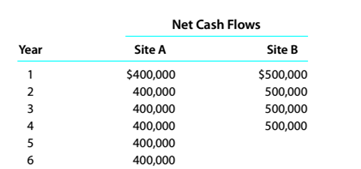Chapter 15, Problem 15.5.3P

Chapter
Section
Textbook Problem

Evaluate alternative capital investment decisionsThe investment committee of Iron Skillet Restaurants Inc. is evaluating two restaurant sites.The sites have different useful lives, hut each requires an investment of $1.000,000. Theestimated net cash flows from each site are as follows:The committee has selected a rate of 20% for purposes of net present value analysis.It also estimates that the residual value at the end of each restaurant’s useful life is$0,but at the end of the fourth year. Site A’s residual value would be $300.000. Instructions Prepare a report to the investment committee, providing your advice on the relative meritsof the two sites. To determine Concept Introduction: NPV: Net present value (NPV) is the method to evaluate the project feasibility. This method calculates the present value of cash inflows and outflows, and then calculates the net present value of the investment. A project should be accepted if it has a positive NPV. The formula to calculate the NPV is as follows: NPV = Present value of cash inflows Present value of cash out flows To Indicate: The Comparison of two sites Explanation The Net present value for each site is calculated as follows:  Site A Net Cash Flows PVF (20%) PV A B =A*B Year 0$ (1,000,000) 1.0000 $(1,000,000) Year 1$ 400,000 0.8333 $333,333 Year 2$ 400,000 0.6944 $277,778 Year 3$ 400,000 0.5787 $231,481 Year 4$ 700,000 0...

Still sussing out bartleby?

Check out a sample textbook solution.

See a sample solution

The Solution to Your Study Problems

Bartleby provides explanations to thousands of textbook problems written by our experts, many with advanced degrees!

Get Started

What is the natural business year?

Financial & Managerial Accounting

Describe how banks create money.

Principles of Macroeconomics (MindTap Course List)

EXPECTATIONS THEORY Assume that the real risk-free rate is 2% and that the maturity risk premium is zero. If a ...

Fundamentals of Financial Management, Concise Edition (with Thomson ONE - Business School Edition, 1 term (6 months) Printed Access Card) (MindTap Course List)

Should an economic model describe reality exactly?

Principles of Microeconomics (MindTap Course List)